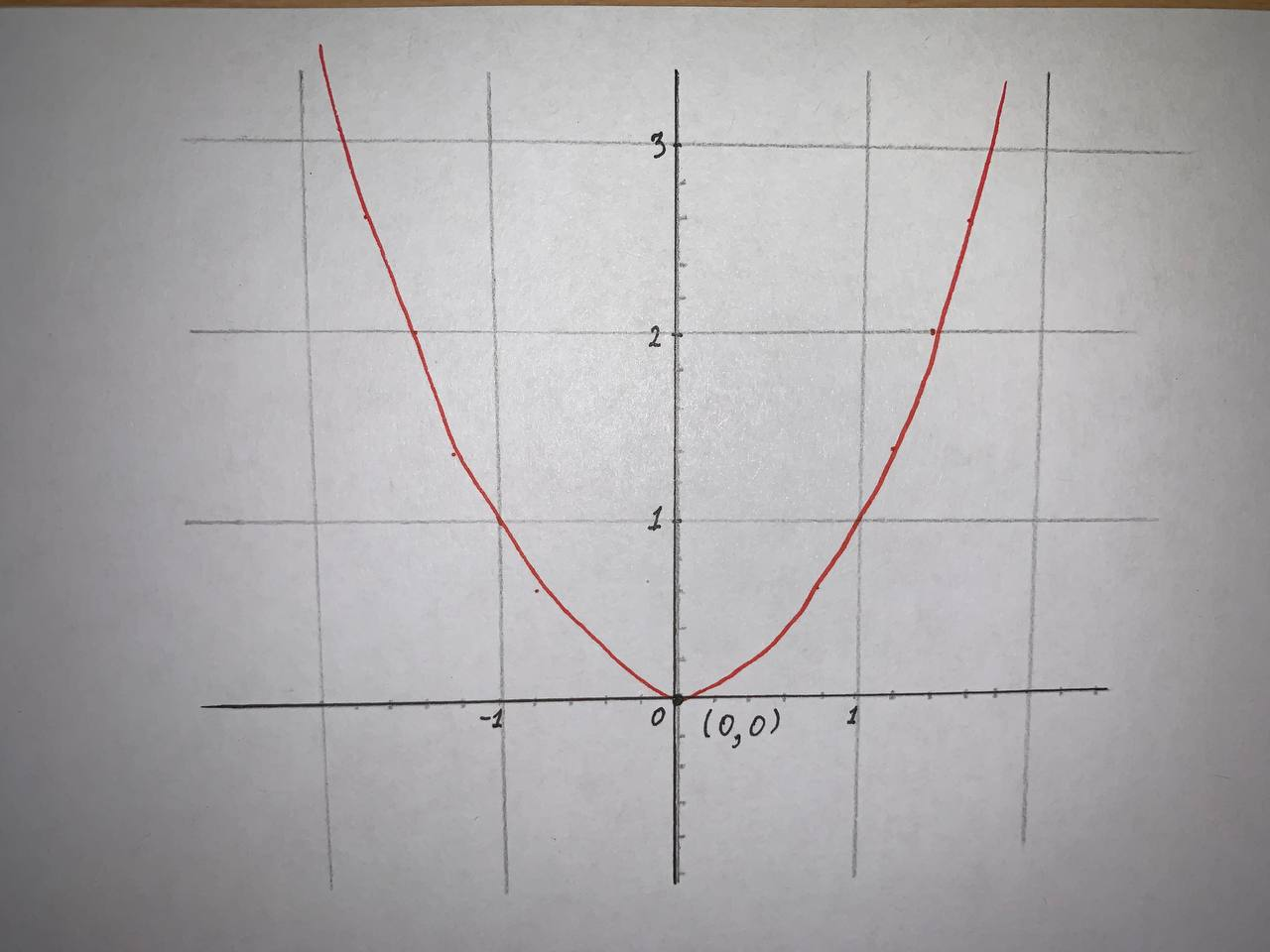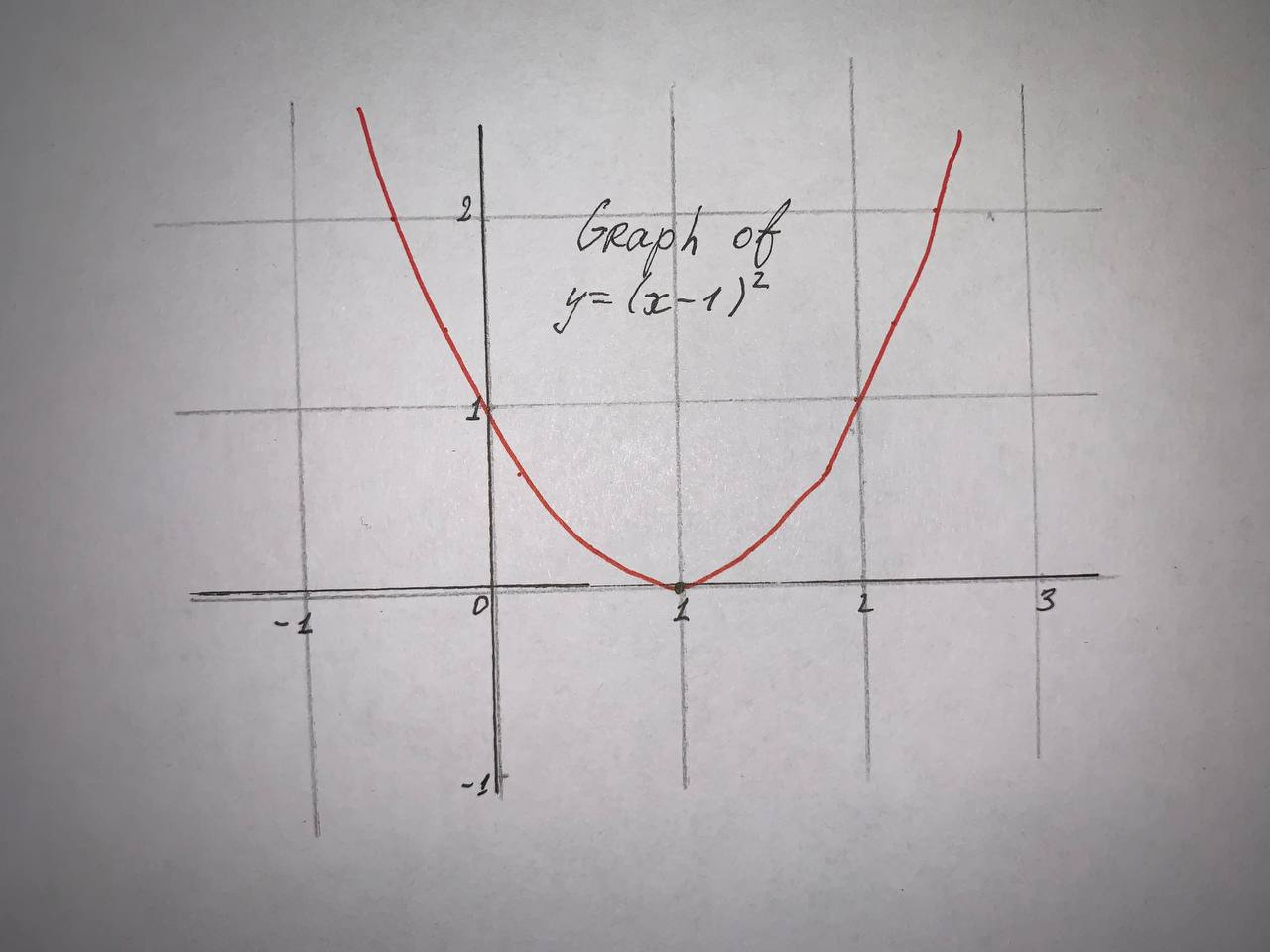Graph the function by hand, not by plotting points, but by starting with the graph of one of the standard functions. y=1-2x+x^2Tobias Ali 2021-09-08 Answered
Graph the function by hand, not by plotting points, but by starting with the graph of one of the standard functions and then applying the appropriate transformations.
$$\displaystyle{y}={1}-{2}{x}+{x}^{{2}}$$

• Questions are typically answered in as fast as 30 minutes

Solve your problem for the price of one coffee

• Math expert for every subject
• Pay only if we can solve itNeelam Wainwright

Step 1
Given:
$$\displaystyle{y}={1}-{2}{x}+{x}^{{2}}$$
To sketch:
The graph of $$\displaystyle{y}={1}-{2}{x}+{x}^{{2}}$$ using transformations.
Step 2
First we need to identify the parent function of $$\displaystyle{y}={1}-{2}{x}+{x}^{{2}}$$
$$\displaystyle{y}={1}-{2}{x}+{x}^{{2}}$$
$$\displaystyle{y}={x}^{{2}}-{2}{x}+{1}$$
$$\displaystyle{y}={\left({x}-{1}\right)}^{{2}}$$
Here the parent function is $$\displaystyle{y}={x}^{{2}}$$ . Using this, we will get the translation graph $$\displaystyle{y}={\left({x}-{1}\right)}^{{2}}$$
Since, $$\displaystyle{y}={\left({x}-{1}\right)}^{{2}}$$ is a parabola with vertex at (1,0) .
Step 3
The graph of $$\displaystyle{y}={x}^{{2}}$$ of vertex (0,0) is given by,The graph of $$y=(x-1)^2$$ is obtained by shifting the graph $$\displaystyle{y}={x}^{{2}}$$ by 1 unit in the right direction.
Thus, the graph of $$\displaystyle{y}={1}-{2}{x}+{x}^{{2}}$$ is given by,